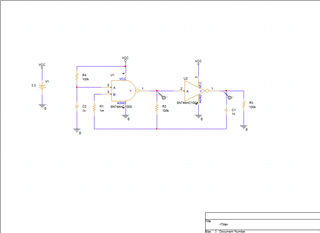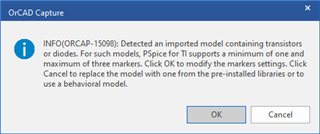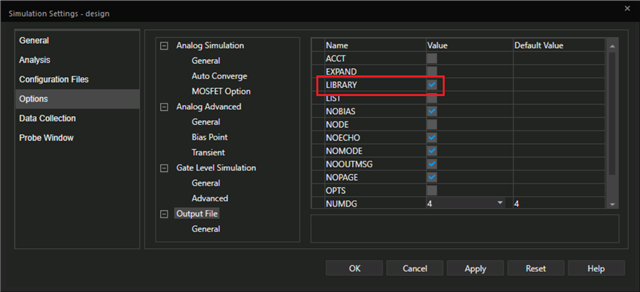# PSPICE-FOR-TI: Simluation Error

Part Number: PSPICE-FOR-TI

Dear,

I received an error infor shown as below.

ERROR(ORPSIM-16583): Detected an imported model containing transistors or diodes. For such models, PSpice for TI supports a minimum of one and maximum of three traces. Reduce the number of traces and simulate again.

How should it be solved?• Hi,

but I did not import any 3rd-party library in this sch. all 74hc series come from the tool itself. and I update the lib timely

• Hi Feng,

would you please share your project with us? You can archive it according to this post: e2e.ti.com/.../faq-pspice-for-ti-how-do-i-share-pspice-for-ti-projects

Thanks,
JC

• Hi Feng,

Thanks for the file. We are looking at this.

Regards,

JC

• Hi Feng,

Do you get this error?

ERROR(ORPSIM-16152): Invalid number: Error while converting token 'maxICC' to double value. Token can be a constant or expression
ERROR(ORPSIM-16152): Invalid number: Error while converting token 'maxICC' to double value. Token can be a constant or expression

Thanks,
JC

• no. below is the error notes.• Hi Feng,

Please turn on the following option and run the simulation again, then share the file "design.out"?Regards,

JC

• design.out.txt
```
**** 07/30/21 22:54:21 ******* PSpice 17.4.0 (Nov 2018) ******* ID# 0 ********

** Profile: "SCHEMATIC1-design"  [ c:\users\cnfezha14\onedrive - abb\.01.work\workspace\ao820\spice\design1-pspicefiles\schematic1\d

****     CIRCUIT DESCRIPTION

******************************************************************************

** Creating circuit file "design.cir"
** WARNING: THIS AUTOMATICALLY GENERATED FILE MAY BE OVERWRITTEN BY SUBSEQUENT SIMULATIONS

*Libraries:
* Profile Libraries :
* Local Libraries :
* From [PSPICE NETLIST] section of C:\cds_spb_home\cdssetup\OrCAD_PSpiceTIPSpice_Install\17.4.0\PSpice.ini file:
.lib "nom_pspti.lib"
.lib "nom.lib"

*Analysis directives:
.TRAN  0 1000ns 0
.OPTIONS LIBRARY
.OPTIONS FILEMODELSEARCH
.PROBE64 V(alias(*)) I(alias(*)) W(alias(*)) D(alias(*)) NOISE(alias(*))
.INC "..\SCHEMATIC1.net"

**** INCLUDING SCHEMATIC1.net ****
* source DESIGN1
X_U1         N00371 N00711 N00424 VCC 0 SN74AHC1G00
X_U2         N00393 N00371 VCC 0 SN74AHC1G04
V_V1         VCC 0 3.3
R_R1         N00400 N00424  1m TC=0,0
R_R2         N00400 N00371  100k TC=0,0
C_C1         N00400 N00393  1n  TC=0,0
R_R3         0 N00393  100k TC=0,0
C_C2         0 N00711  1n  TC=0,0
R_R4         N00711 VCC  100k TC=0,0

**** RESUMING design.cir ****
.END

**** FROM LIBRARY SN74AHC1G00.lib ****
.SUBCKT SN74AHC1G00 Y A B VCC AGND
XU1 Y A B VCC AGND LOGIC_GATE_2PIN_OD_LVC_2i_NAND_PP_CMOS_SN74AHC1G00
.ENDS

**** FROM LIBRARY SN74AHC1G04.lib ****
.SUBCKT SN74AHC1G04 Y A VCC AGND
XU1 Y A VCC VCC AGND LOGIC_GATE_2PIN_OD_AHC_1i_NAND_PP_CMOS_SN74AHC1G04
.ENDS

**** FROM LIBRARY SN74AHC1G00.lib ****
.SUBCKT LOGIC_GATE_2PIN_OD_LVC_2i_NAND_PP_CMOS_SN74AHC1G00 OUT A B VCC GND

.PARAM VCC_ABS_MAX = 7
.PARAM VCC_MAX = 5.5
.PARAM RA = 2200000000
.PARAM RB = 2200000000
.PARAM CA = 1e-11
.PARAM CB = 1e-11
.PARAM ROEZ = 2000.0000000000016
.PARAM COEZ = 1e-11
RA  A  GND {RA}
RB  B  GND {RB}
CA  A  GND {CA}
CB  B  GND {CB}
XUA NA A VCC GND LOGIC_INPUT_LVC_2i_NAND_PP_CMOS_SN74AHC1G00
XUB NB B VCC GND LOGIC_INPUT_LVC_2i_NAND_PP_CMOS_SN74AHC1G00
XUG NA NB NOUTG VCC GND LOGIC_FUNCTION_2_LVC_2i_NAND_PP_CMOS_SN74AHC1G00
XOUTPD NOUTG NOUTTPD VCC GND TPD_LVC_2i_NAND_PP_CMOS_SN74AHC1G00
XUOUT NOUTTPD NOUT_INT VCC GND LOGIC_PP_OUTPUT_LVC_2i_NAND_PP_CMOS_SN74AHC1G00
XICC VCC GND NVIOUT LOGIC_ICC_LVC_2i_NAND_PP_CMOS_SN74AHC1G00
SICC VCC GND VCC GND SW1
H1 NVIOUT GND VIOUT 1
VIOUT NOUT_INT OUTsw 0
SIOFF OUTsw OUT VCC GND SW2
DA2 GND A D1
DB2 GND B D1
DO2 GND OUT D1
RDA1 NA1 GND 1e6
SDA1 NA1 A VCC GND SW2
RDB1 NB1 GND 1e6
SDB1 NB1 B VCC GND SW2
RDO1 NO1 GND 1e6
SDO1 NO1 OUT VCC GND SW2
.MODEL SW1 VSWITCH VON = {VCC_ABS_MAX} VOFF = {VCC_MAX} RON = 10 ROFF = 60e6
.MODEL SW2 VSWITCH VON = {0.55} VOFF = {0.45} RON = 10m ROFF = 100e6
.MODEL D1 D
.ENDS

**** FROM LIBRARY SN74AHC1G04.lib ****
.SUBCKT LOGIC_GATE_2PIN_OD_AHC_1i_NAND_PP_CMOS_SN74AHC1G04 OUT A B VCC GND

.PARAM VCC_ABS_MAX = 7
.PARAM VCC_MAX = 5.5
.PARAM RA = 880000000
.PARAM RB = 880000000
.PARAM CA = 1e-11
.PARAM CB = 1e-11
.PARAM ROEZ = 2000
.PARAM COEZ = 3e-12
RA  A  GND {RA}
RB  B  GND {RB}
CA  A  GND {CA}
CB  B  GND {CB}
XUA NA A VCC GND LOGIC_INPUT_AHC_1i_NAND_PP_CMOS_SN74AHC1G04
XUB NB B VCC GND LOGIC_INPUT_AHC_1i_NAND_PP_CMOS_SN74AHC1G04
XUG NA NB NOUTG VCC GND LOGIC_FUNCTION_2_AHC_1i_NAND_PP_CMOS_SN74AHC1G04
XOUTPD NOUTG NOUTTPD VCC GND TPD_AHC_1i_NAND_PP_CMOS_SN74AHC1G04
XUOUT NOUTTPD NOUT_INT VCC GND LOGIC_PP_OUTPUT_AHC_1i_NAND_PP_CMOS_SN74AHC1G04
XICC VCC GND NVIOUT LOGIC_ICC_AHC_1i_NAND_PP_CMOS_SN74AHC1G04
SICC VCC GND VCC GND SW1
H1 NVIOUT GND VIOUT 1
VIOUT NOUT_INT OUTsw 0
SIOFF OUTsw OUT VCC GND SW2
DA2 GND A D1
DB2 GND B D1
DO2 GND OUT D1
RDA1 NA1 GND 1e6
SDA1 NA1 A VCC GND SW2
RDB1 NB1 GND 1e6
SDB1 NB1 B VCC GND SW2
RDO1 NO1 GND 1e6
SDO1 NO1 OUT VCC GND SW2
.MODEL SW1 VSWITCH VON = {VCC_ABS_MAX} VOFF = {VCC_MAX} RON = 10 ROFF = 60e6
.MODEL SW2 VSWITCH VON = {0.55} VOFF = {0.45} RON = 10m ROFF = 100e6
.MODEL D1 D
.ENDS

**** FROM LIBRARY SN74AHC1G00.lib ****
.SUBCKT LOGIC_INPUT_LVC_2i_NAND_PP_CMOS_SN74AHC1G00 OUT IN VCC VEE
.PARAM STANDARD_INPUT_SELECT = 1

.PARAM SCHMITT_TRIGGER_INPUT_SELECT = 0
ESTD_THR VSTD_THR VEE TABLE {V(VCC,VEE)} =
+(1,0.5)
+(1.8,0.9)
+(2.5,1.25)
+(3.3,1.65)
+(5,2.5)
+(6,3)
ETRP_P VTRP_P VEE TABLE {V(VCC,VEE)} =
+(3,1.2)
+(4.5,1.75)
+(5.5,2.15)
ETRP_N VTRP_N VEE TABLE {V(VCC,VEE)} =
+(3,0.9)
+(4.5,1.35)
+(5.5,1.65)
EHYST VHYST VEE TABLE {V(VCC,VEE)} =
+(3,0.3)
+(4.5,0.4)
+(5.5,0.5)
ETRUE NTRUE VEE VALUE = {V(VCC,VEE)}
EFALSE NFALSE VEE VALUE = {0}

EBETA BETA VEE VALUE = {V(VHYST,VEE)/(V(NTRUE,VEE) - V(NFALSE,VEE) + V(VHYST,VEE))}
EFB NFB VEE VALUE = {(1 - V(BETA,VEE))*V(IN,VEE) + V(BETA,VEE)*V(CURR_OUT,VEE)}
EREF NREF VEE VALUE = {0.5*(1 - V(BETA,VEE))*(V(VTRP_P,VEE) + V(VTRP_N,VEE))
+ + 0.5*V(BETA,VEE)*(V(NTRUE,VEE) + V(NFALSE,VEE))}
EDIFF NDIFF VEE VALUE = {V(NFB,NREF)}
ESWITCH VSWITCH VEE VALUE = {0.5*(-SGN(V(NDIFF,VEE)) + ABS(SGN(V(NDIFF,VEE))))}
ESWITCH1 VSWITCH1 VEE VALUE = {0.5*(SGN(V(NDIFF,VEE)) + ABS(SGN(V(NDIFF,VEE))))}
GCOMP VEE CURR_OUT VALUE = {SCHMITT_TRIGGER_INPUT_SELECT*0.5*V(VCC,VEE)*(SGN(V(NDIFF,VEE)) + ABS(SGN(V(NDIFF,VEE))))}

GSTD VEE CURR_OUT VALUE = {STANDARD_INPUT_SELECT*0.5*V(VCC,VEE)*(SGN(V(IN,VSTD_THR)) + ABS(SGN(V(IN,VSTD_THR))))}
ROUT CURR_OUT VEE 1
EMID MID VEE VALUE = {0.5*(V(VCC,VEE) + V(VEE))}
EARG NARG VEE VALUE = {V(CURR_OUT,VEE) - V(MID,VEE)}
EOUT OUT VEE VALUE = {0.5*(SGN(V(NARG,VEE)) + ABS(SGN(V(NARG,VEE) ) ) )}

.PARAM MAXICC = .0009
.PARAM VT = .7
.PARAM VCC_MIN = 3

EV_VT1 VTN VEE VALUE = { VT }
EV_VT2 VTP VEE VALUE = { V(VCC,VEE) - VT }

ETEST TEST VEE VALUE = {.9*V(VCC,VEE)}

EVTHDIFF VTH_DIFF VEE VALUE = {V(IN,VSTD_THR)}
EVTHPDIFF VTHP_DIFF VEE VALUE = {V(IN,VTRP_P)}
EVTHNDIFF VTHN_DIFF VEE VALUE = {V(IN,VTRP_N)}
EVTNDIFF VTN_DIFF VEE VALUE = { V(IN,VTN) }
EVTPDIFF VTP_DIFF VEE VALUE = { V(IN,VTP) }

GICCVA VCC VEE VALUE = { (-ABS(( (1+SGN(V(VTN_DIFF,VEE)) ) )/2 -1) *
+ 2*MAXICC*((V(IN,VEE)-VT)/V(VCC,VEE))^2)*(1 + SGN(V(VCC,VEE) - VCC_MIN))*V(VSWITCH,VEE)}
GICCVB VCC VEE VALUE = { (ABS(( (1+SGN(V(VTHP_DIFF,VEE)) ) )/2 -1) *
+ 2*MAXICC*((V(IN,VEE)-VT)/V(VCC,VEE))^2)*(1 + SGN(V(VCC,VEE) - VCC_MIN))*V(VSWITCH,VEE)}
GICCVC VCC VEE VALUE = { ( ABS(  (1+SGN(V(VTHN_DIFF,VEE)) ) )/2  *
+ 2*MAXICC*((V(IN,VEE)-(V(VCC,VEE)-VT))/V(VCC,VEE))^2)*(1 + SGN(V(VCC,VEE) - VCC_MIN))*V(VSWITCH1,VEE)}
GICCVD VCC VEE VALUE = { (-ABS(  (1+SGN(V(VTP_DIFF,VEE)) ) )/2  *
+ 2*MAXICC*((V(IN,VEE)-(V(VCC,VEE)-VT))/V(VCC,VEE))^2)*(1 + SGN(V(VCC,VEE) - VCC_MIN))*V(VSWITCH1,VEE)}

.ENDS

.SUBCKT LOGIC_FUNCTION_2_LVC_2i_NAND_PP_CMOS_SN74AHC1G00 A B OUT VCC VEE
.PARAM AND  = 0
.PARAM NAND = 1
.PARAM OR   = 0
.PARAM NOR  = 0
.PARAM XOR  = 0
.PARAM XNOR = 0
GAND  VEE N1 VALUE = {AND*V(A,VEE)*V(B,VEE)}
GNAND VEE N1 VALUE = {NAND*(1 - V(A,VEE)*V(B,VEE))}
GOR   VEE N1 VALUE = {OR*(MIN(V(A,VEE) + V(B,VEE),1))}
GNOR  VEE N1 VALUE = {NOR*(1 - MIN(V(A,VEE) + V(B,VEE),1))}
GXOR  VEE N1 VALUE = {XOR*((1 - V(A,VEE))*V(B,VEE) + V(A,VEE)*(1 - V(B,VEE)))}
GXNOR VEE N1 VALUE = {XNOR*(1 - ((1 - V(A,VEE))*V(B,VEE) + V(A,VEE)*(1 - V(B,VEE))))}
RN1 N1 VEE 1
EOUT OUT VEE N1 VEE 1
.ENDS

.SUBCKT TPD_LVC_2i_NAND_PP_CMOS_SN74AHC1G00 IN OUT VCC VEE
.PARAM TPDELAY1 = 1N
.PARAM RS = 10K
.PARAM CS = {-TPDELAY1/(RS*LOG(0.5))}
ETPDNORM NTPDNORM VEE TABLE {V(VCC,VEE)} =
+(3.3,6.7)
+(5,4.7)
G1 IN N1 VALUE = {V(IN,N1)/(V(NTPDNORM,VEE)*RS)}
RZ IN N1 10G
C1 N1 VEE {CS}
E1 N2 VEE VALUE = {0.5*(1 + SGN(V(N1,VEE) - 0.5))}
EOUT OUT VEE N2 VEE 1
.ENDS

.SUBCKT LOGIC_PP_OUTPUT_LVC_2i_NAND_PP_CMOS_SN74AHC1G00 IN OUT VCC VEE
EROH NROH VEE TABLE {V(VCC,VEE)} =
+(2,2000)
+(3,105)
+(4.5,70)
EROL NROL VEE TABLE {V(VCC,VEE)} =
+(2,2000)
+(3,90)
+(4.5,46.25)
E1 N1 VEE VALUE = {V(VCC,VEE)*V(IN,VEE)}
GOUT N1 OUT VALUE = {V(N1,OUT)*(V(IN,VEE)/V(NROH,VEE) + (1 - V(IN,VEE))/V(NROL,VEE))}
.ENDS

.SUBCKT LOGIC_ICC_LVC_2i_NAND_PP_CMOS_SN74AHC1G00 VCC VEE VIOUT
.PARAM ICC = 5e-09
.PARAM VCC_MAX = 5.5
.PARAM VCC_MIN = 2
GICC VCC VEE VALUE = {ICC*0.5*(1 + SGN(V(VCC,VEE) - VCC_MIN))}
EGNDF GNDF 0 VALUE = {0.5*(V(VCC) + V(VEE))}
GOUTP VCC GNDF VALUE = {V(VIOUT,VEE)*0.5*(SGN(V(VIOUT,VEE)) + ABS(SGN(V(VIOUT,VEE))))}
GOUTN GNDF VEE VALUE = {V(VIOUT,VEE)*0.5*(SGN(V(VIOUT,VEE)) + ABS(SGN(V(VIOUT,VEE))))}
.ENDS

**** FROM LIBRARY SN74AHC1G04.lib ****
.SUBCKT LOGIC_INPUT_AHC_1i_NAND_PP_CMOS_SN74AHC1G04 OUT IN VCC VEE
.PARAM STANDARD_INPUT_SELECT = 1

.PARAM SCHMITT_TRIGGER_INPUT_SELECT = 0
ESTD_THR VSTD_THR VEE TABLE {V(VCC,VEE)} =
+(1,0.5)
+(1.8,0.9)
+(2.5,1.25)
+(3.3,1.65)
+(5,2.5)
+(6,3)
ETRP_P VTRP_P VEE TABLE {V(VCC,VEE)} =
+(4.5,1.5)
+(5.5,1.6)
ETRP_N VTRP_N VEE TABLE {V(VCC,VEE)} =
+(4.5,0.7)
+(5.5,0.8)
EHYST VHYST VEE TABLE {V(VCC,VEE)} =
+(4.5,0.8)
+(5.5,0.8)
ETRUE NTRUE VEE VALUE = {V(VCC,VEE)}
EFALSE NFALSE VEE VALUE = {0}
EBETA BETA VEE VALUE = {V(VHYST,VEE)/(V(NTRUE,VEE) - V(NFALSE,VEE) + V(VHYST,VEE))}
EFB NFB VEE VALUE = {(1 - V(BETA,VEE))*V(IN,VEE) + V(BETA,VEE)*V(CURR_OUT,VEE)}
EREF NREF VEE VALUE = {0.5*(1 - V(BETA,VEE))*(V(VTRP_P,VEE) + V(VTRP_N,VEE))
+ + 0.5*V(BETA,VEE)*(V(NTRUE,VEE) + V(NFALSE,VEE))}
EDIFF NDIFF VEE VALUE = {V(NFB,NREF)}
ESWITCH VSWITCH VEE VALUE = {0.5*(-SGN(V(NDIFF,VEE)) + ABS(SGN(V(NDIFF,VEE))))}
ESWITCH1 VSWITCH1 VEE VALUE = {0.5*(SGN(V(NDIFF,VEE)) + ABS(SGN(V(NDIFF,VEE))))}
GCOMP VEE CURR_OUT VALUE = {SCHMITT_TRIGGER_INPUT_SELECT*0.5*V(VCC,VEE)*(SGN(V(NDIFF,VEE)) + ABS(SGN(V(NDIFF,VEE))))}
GSTD VEE CURR_OUT VALUE = {STANDARD_INPUT_SELECT*0.5*V(VCC,VEE)*(SGN(V(IN,VSTD_THR)) + ABS(SGN(V(IN,VSTD_THR))))}
ROUT CURR_OUT VEE 1
EMID MID VEE VALUE = {0.5*(V(VCC,VEE) + V(VEE))}
EARG NARG VEE VALUE = {V(CURR_OUT,VEE) - V(MID,VEE)}
EOUT OUT VEE VALUE = {0.5*(SGN(V(NARG,VEE)) + ABS(SGN(V(NARG,VEE) ) ) )}
.PARAM MAXICC = .0009
.PARAM VT = .7
.PARAM VCC_MIN = 2

EV_VT1 VTN VEE VALUE = { VT }
EV_VT2 VTP VEE VALUE = { V(VCC,VEE) - VT }

ETEST TEST VEE VALUE = {.9*V(VCC,VEE)}

EVTHDIFF VTH_DIFF VEE VALUE = {V(IN,VSTD_THR)}
EVTHPDIFF VTHP_DIFF VEE VALUE = {V(IN,VTRP_P)}
EVTHNDIFF VTHN_DIFF VEE VALUE = {V(IN,VTRP_N)}
EVTNDIFF VTN_DIFF VEE VALUE = { V(IN,VTN) }
EVTPDIFF VTP_DIFF VEE VALUE = { V(IN,VTP) }

GICCVA VCC VEE VALUE = { (-ABS(( (1+SGN(V(VTN_DIFF,VEE)) ) )/2 -1) *
+ 2*MAXICC*((V(IN,VEE)-VT)/V(VCC,VEE))^2)*(1 + SGN(V(VCC,VEE) - VCC_MIN))*V(VSWITCH,VEE)}
GICCVB VCC VEE VALUE = { (ABS(( (1+SGN(V(VTHP_DIFF,VEE)) ) )/2 -1) *
+ 2*MAXICC*((V(IN,VEE)-VT)/V(VCC,VEE))^2)*(1 + SGN(V(VCC,VEE) - VCC_MIN))*V(VSWITCH,VEE)}
GICCVC VCC VEE VALUE = { ( ABS(  (1+SGN(V(VTHN_DIFF,VEE)) ) )/2  *
+ 2*MAXICC*((V(IN,VEE)-(V(VCC,VEE)-VT))/V(VCC,VEE))^2)*(1 + SGN(V(VCC,VEE) - VCC_MIN))*V(VSWITCH1,VEE)}
GICCVD VCC VEE VALUE = { (-ABS(  (1+SGN(V(VTP_DIFF,VEE)) ) )/2  *
+ 2*MAXICC*((V(IN,VEE)-(V(VCC,VEE)-VT))/V(VCC,VEE))^2)*(1 + SGN(V(VCC,VEE) - VCC_MIN))*V(VSWITCH1,VEE)}

.ENDS

.SUBCKT LOGIC_FUNCTION_2_AHC_1i_NAND_PP_CMOS_SN74AHC1G04 A B OUT VCC VEE
.PARAM AND  = 0
.PARAM NAND = 1
.PARAM OR   = 0
.PARAM NOR  = 0
.PARAM XOR  = 0
.PARAM XNOR = 0
GAND  VEE N1 VALUE = {AND*V(A,VEE)*V(B,VEE)}
GNAND VEE N1 VALUE = {NAND*(1 - V(A,VEE)*V(B,VEE))}
GOR   VEE N1 VALUE = {OR*(MIN(V(A,VEE) + V(B,VEE),1))}
GNOR  VEE N1 VALUE = {NOR*(1 - MIN(V(A,VEE) + V(B,VEE),1))}
GXOR  VEE N1 VALUE = {XOR*((1 - V(A,VEE))*V(B,VEE) + V(A,VEE)*(1 - V(B,VEE)))}
GXNOR VEE N1 VALUE = {XNOR*(1 - ((1 - V(A,VEE))*V(B,VEE) + V(A,VEE)*(1 - V(B,VEE))))}
RN1 N1 VEE 1
EOUT OUT VEE N1 VEE 1
.ENDS

.SUBCKT TPD_AHC_1i_NAND_PP_CMOS_SN74AHC1G04 IN OUT VCC VEE
.PARAM TPDELAY1 = 1N
.PARAM RS = 10K
.PARAM CS = {-TPDELAY1/(RS*LOG(0.5))}
ETPDNORM NTPDNORM VEE TABLE {V(VCC,VEE)} =
+(3.3,5.75)
+(5,4)
G1 IN N1 VALUE = {V(IN,N1)/(V(NTPDNORM,VEE)*RS)}
RZ IN N1 10G
C1 N1 VEE {CS}
E1 N2 VEE VALUE = {0.5*(1 + SGN(V(N1,VEE) - 0.5))}
EOUT OUT VEE N2 VEE 1
.ENDS

.SUBCKT LOGIC_PP_OUTPUT_AHC_1i_NAND_PP_CMOS_SN74AHC1G04 IN OUT VCC VEE
EROH NROH VEE TABLE {V(VCC,VEE)} =
+(2,0)
+(3,63)
+(4.5,42)
EROL NROL VEE TABLE {V(VCC,VEE)} =
+(2,1200)
+(3,54)
+(4.5,27.75)
E1 N1 VEE VALUE = {V(VCC,VEE)*V(IN,VEE)}
GOUT N1 OUT VALUE = {V(N1,OUT)*(V(IN,VEE)/V(NROH,VEE) + (1 - V(IN,VEE))/V(NROL,VEE))}
.ENDS

.SUBCKT LOGIC_ICC_AHC_1i_NAND_PP_CMOS_SN74AHC1G04 VCC VEE VIOUT
.PARAM ICC = 1.25e-07
.PARAM VCC_MAX = 5.5
.PARAM VCC_MIN = 2
GICC VCC VEE VALUE = {ICC*0.5*(1 + SGN(V(VCC,VEE) - VCC_MIN))}
EGNDF GNDF 0 VALUE = {0.5*(V(VCC) + V(VEE))}
GOUTP VCC GNDF VALUE = {V(VIOUT,VEE)*0.5*(SGN(V(VIOUT,VEE)) + ABS(SGN(V(VIOUT,VEE))))}
GOUTN GNDF VEE VALUE = {V(VIOUT,VEE)*0.5*(SGN(V(VIOUT,VEE)) + ABS(SGN(V(VIOUT,VEE))))}
.ENDS

**** 07/30/21 22:54:21 ******* PSpice 17.4.0 (Nov 2018) ******* ID# 0 ********

** Profile: "SCHEMATIC1-design"  [ c:\users\cnfezha14\onedrive - abb\.01.work\workspace\ao820\spice\design1-pspicefiles\schematic1\d

****     Diode MODEL PARAMETERS

******************************************************************************

X_U1.XU1.D1     X_U2.XU1.D1
IS   10.000000E-15   10.000000E-15

**** 07/30/21 22:54:21 ******* PSpice 17.4.0 (Nov 2018) ******* ID# 0 ********

** Profile: "SCHEMATIC1-design"  [ c:\users\cnfezha14\onedrive - abb\.01.work\workspace\ao820\spice\design1-pspicefiles\schematic1\d

****     Voltage Controlled Switch MODEL PARAMETERS

******************************************************************************

X_U1.XU1.SW1    X_U1.XU1.SW2    X_U2.XU1.SW1    X_U2.XU1.SW2
RON   10                .01           10                .01
ROFF   60.000000E+06  100.000000E+06   60.000000E+06  100.000000E+06
VON    7                .55            7                .55
VOFF    5.5              .45            5.5              .45

ERROR(ORPSIM-16583): Detected an imported model containing transistors or diodes. For such models, PSpice for TI supports a minimum of one and maximum of three traces. Reduce the number of traces and simulate again.

ABORTING SIMULATION
**** 07/30/21 22:54:21 ******* PSpice 17.4.0 (Nov 2018) ******* ID# 0 ********

** Profile: "SCHEMATIC1-design"  [ c:\users\cnfezha14\onedrive - abb\.01.work\workspace\ao820\spice\design1-pspicefiles\schematic1\d

****     JOB STATISTICS SUMMARY

******************************************************************************

Node counts:
Top level (NUNODS)                =           7
External (NCNODS)                 =         135
Total (NUMNOD)                    =         135

Total device count (NUMEL)          =         205
Capacitors (C)                    =           8
Diodes (D)                        =           6
VCVS (E)                          =         108
VCCS (G)                          =          46
CCVS (H)                          =           2
Resistors (R)                     =          22
VSwitches (S)                     =          10
Voltage Sources (V)               =           3

Number of subcircuits (X)           =          16

Matrix statistics:
Matrix size (NSTOP)               =         248
Initial no. elements (NTTAR)      =         535
No. elements w/ fillin (NTTBR)    =         535
No. fillins (IFILL)               =           0
No. overflows (NTTOV)             =           0
No. LU operations (IOPS)          =           0
Percent sparsity (PERSPA)         =      99.130

Analysis statistics:
No. total time points (NUMTTP)    =           0
No. rejected time points (NUMRTP) =           0
No. iterations (NUMNIT)           =           0

Runtime statistics:                       Seconds      Iterations
Matrix solution                   =        0.00               1